# Soup from canteen

For how many people is 90 liters of soup enough if we assume 3/8 liter of soup per person in the canteen?

Correct result:

n =  240

#### Solution: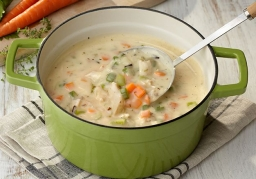We would be pleased if you find an error in the word problem, spelling mistakes, or inaccuracies and send it to us. Thank you!Tips to related online calculators
Need help calculate sum, simplify or multiply fractions? Try our fraction calculator.
Tip: Our volume units converter will help you with the conversion of volume units.
Do you want to round the number?

## Next similar math problems:

• InqualitySolve inequality: 3x + 6 > 14
• Equation with xSolve the following equation: 2x- (8x + 1) - (x + 2) / 5 = 9
• UN 1If we add to an unknown number his quarter, we get 210. Identify unknown number.
• Orchard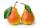Three-fifths of the orchard are apple trees, one-third of the trees are cherries. The remaining 5 trees are pears. How many trees are in the orchard?
• Equation with fractionsSolve equation: ? It is equation with fractions.
• Cleaning windowsCleaning company has to wash all the windows of the school. The first day washes one-sixth of the windows of the school, the next day three more windows than the first day and the remaining 18 windows washes on the third day. Calculate how many windows ha
• Tank of fuelA 14.5-gallon tank of fuel is 3/4 full. How many more gallons will it take to fill up the tank?
• Mixing 5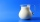Carlos mixed 4/15 of chocolate syrup with 1/2 of milk. Determine the reasonable estimate of the total amount of liquid
• Percent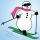From 99 children who participated in the ski course were 23 excellent skiers, 13 good and 20 average and the rest were beginners. Calculate this data in percentages.
• Bag of peanuts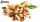Joe eat 1/3 of a bag of peanuts, mark eat 1/4 of the remaining in the bag of peanuts, Alvin eat 1/2 of the remaining bag of peanuts, peter eat 10 peanuts, there are 71 peanuts left. Hon many peanuts were in the bags?
• Find the 11Find the quotient of 229.12 and 12.32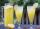How many 1/4 cup servings are in 2 and 1/3 cups of lemonade?Calculate 61% if 85% is 38.Divide the number 72 in the ratio 7: 2 and calculate the ratio of the numbers found in this order and write down as decimal.A vehicle tank was 3/5 full of petrol. When 21 liters of fuel was added it was 5/6 full. How many liters of petrol can the tank hold?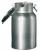How many three-quarters liters jar fill 30 liters container?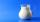At the kindergarten, every child got 1/5 liter of milk in the morning and another 1/8 liter of milk in the afternoon. How many liters were consumed per day for 20 children?